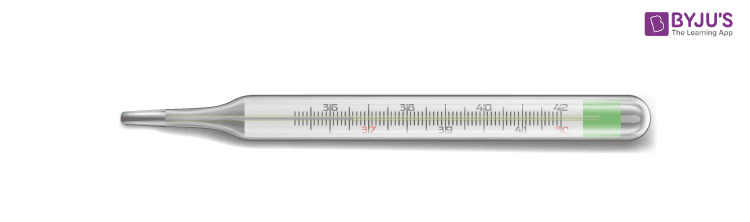# Temperature Measurement ThermometerTemperature measurement refers to the process of obtaining the current temperature locally for immediate requirements or for later. Temperature measurement is required in a variety of fields, from metallurgy to baking, from your kitchen to multi-billion dollar industries. There is a wide array of needs in the process control industry and to meet these needs, there has been a development of numerous sensors and devices. So before we begin with temperature measurement let’s take a good look at temperature. A common instrument used for the measurement of temperature is known as the thermometer

## Temperature Sensors: ThermometerThe thermometer is the oldest form of a temperature sensor, although the first working model came about very late. The first thermometers were designed in the 16th century and unlike how we remember the thermometer, it was filled with gas. The current design of a hollow cylindrical tube filled with mercury was conceived and perfected by Daniel Gabriel Fahrenheit, who chose the metal mercury for two vital reasons. Mercury does not stick to the glass tube and irrespective of the temperature exhibits an equal rate of thermal expansion.

Thermal expansion is a phenomenon we will thoroughly address later. The expansion of mercury due to heat is what tells us the temperature. If we calculate the expansion of mercury per degree change in temperature; then we can calibrate the thermometers with a scale based on the expansion. This is how a thermometer works. In honour of Fahrenheit’s discovery, we have named the temperature scale after him.

The temperature sensors are so calibrated that the numbers on the thermometers represent the temperature of the body. Now we know that for any scale, we need the two reference points. The most obvious and sufficiently stable reference points are the boiling point and the melting point of water; being 100o C and 0oC. At 100, the water boils over into the vapour state and at 0, the water melts from solid to a liquid state.

On the Fahrenheit scale, this would turn out to be 212oF for the boiling point and 32oF for the melting point. The Fahrenheit scale is used in North America and a few other countries and the Celsius scale is used by the rest of the world. Below you will find the formulae for its conversion;

$$T_{c}$$ =$$(T_{f} – 32).\frac{5}{9}$$
Test Your Knowledge On Temperature Measurement Thermometer!